# GMAT Math : DSQ: Understanding arithmetic sets

## Example Questions

← Previous 1

### Example Question #1 : Sets

How many subsets does sethave?

Statement 1:has eight elements.

Statement 2:is the set of all prime numbers between 1 and 20.

Statement 1 ALONE is sufficient to answer the question, but Statement 2 ALONE is NOT sufficient to answer the question.

BOTH statements TOGETHER are insufficient to answer the question.

EITHER statement ALONE is sufficient to answer the question.

Statement 2 ALONE is sufficient to answer the question, but Statement 1 ALONE is NOT sufficient to answer the question.

BOTH statements TOGETHER are sufficient to answer the question, but NEITHER statement ALONE is sufficient to answer the question.

EITHER statement ALONE is sufficient to answer the question.

Explanation:

The number of subsets of any set can be calculated by raising 2 to the power of the number of elements in the set. The first statement gives you that information immediately. The second gives you enough information to find the number of elements, as there are eight primes between 1 and 20: 2, 3, 5, 7, 11, 13, 17, and 19. From either statement alone, you can deduce the answer to be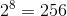.

### Example Question #1 : Dsq: Understanding Arithmetic Sets

Letbe the set of all of the multiples of 3 between 29 and 50. How many subsets ofcan be formed?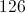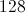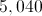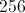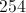Explanation:

The multiples of 3 between 29 and 50 are 30, 33, 36, 39, 42, 45, and 48 - therefore,has seven elements total.

The number of subsets in a set can be calculated by raising 2 to the power of the number of elements. Therefore, the answer to our question is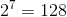.

### Example Question #3 : Sets

The senior class of Watson High School has 613 students. An election was held for Senior Class President between three candidates, Martindale, Nance, and Osgood.

If every student voted for one of these three, and the student with the most votes was declared the winner, who won the election?

Statement 1: Martindale got 240 votes.

Statement 2: Nance got 244 votes.

EITHER statement ALONE is sufficient to answer the question.

BOTH statements TOGETHER are insufficient to answer the question.

BOTH statements TOGETHER are sufficient to answer the question, but NEITHER statement ALONE is sufficient to answer the question.

Statement 1 ALONE is sufficient to answer the question, but Statement 2 ALONE is NOT sufficient to answer the question.

Statement 2 ALONE is sufficient to answer the question, but Statement 1 ALONE is NOT sufficient to answer the question.

BOTH statements TOGETHER are sufficient to answer the question, but NEITHER statement ALONE is sufficient to answer the question.

Explanation:

If we only know Martindale got 240 votes, since 240 is not a majority, we cannot determine the winner with certainty. For example, the following two results are possible:

Martindale: 240, Nance: 0, Osgood 373 - Osgood wins

Martindale 240, Nance 373, Osgood 0 - Nance wins.

A similar argument shows the second statement insufficient as well, since 244 is not a majority.

But the two statements together allow us a complete picture;

Martindale 240, Nance 244, Osgood 129 - Nance wins

### Example Question #4 : Sets

The senior class has 457 students. An election was held for Senior Class President between three candidates, Anderson, Benson, and Carter.

If every student voted for one of these three, and the student with the most votes was declared the winner, who won the election?

Statement 1: Benson got 251 votes.

Statement 2: Carter got 101 votes.

Statement 1 ALONE is sufficient to answer the question, but Statement 2 ALONE is NOT sufficient to answer the question.

EITHER statement ALONE is sufficient to answer the question.

BOTH statements TOGETHER are insufficient to answer the question.

BOTH statements TOGETHER are sufficient to answer the question, but NEITHER statement ALONE is sufficient to answer the question.

Statement 2 ALONE is sufficient to answer the question, but Statement 1 ALONE is NOT sufficient to answer the question.

Statement 1 ALONE is sufficient to answer the question, but Statement 2 ALONE is NOT sufficient to answer the question.

Explanation:

From Statement 1 alone, it can be immediately deduced that Benson won, since 251 of the 457 votes consititute a majority: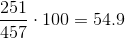Benson got 54.9% of the vote, so there is no way that Anderson or Carter could have bested Benson.

Statement 2 tells only that Carter did not win, since either Anderson or Benson had to have won at least half, or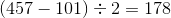; we cannot, however, tell which one it was without further information.

### Example Question #1 : Dsq: Understanding Arithmetic Sets

Let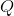be the set of all perfect squares and perfect cubes between 1 and 100 inclusive. How many subsets doeshave?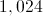Infinitely many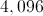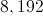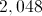Explanation: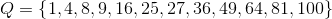, which is a set of 12 elements. A set this size has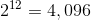subsets.

### Example Question #6 : Sets

Given that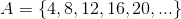and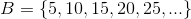, is it true that positive integer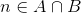?

Statement 1: The last digit ofis a 0.

Statement 2: The second-to-last digit ofis 5.

Statement 1 ALONE is sufficient to answer the question, but Statement 2 ALONE is NOT sufficient to answer the question.

BOTH statements TOGETHER are sufficient to answer the question, but NEITHER statement ALONE is sufficient to answer the question.

BOTH statements TOGETHER are insufficient to answer the question.

Statement 2 ALONE is sufficient to answer the question, but Statement 1 ALONE is NOT sufficient to answer the question.

EITHER statement ALONE is sufficient to answer the question.

Statement 2 ALONE is sufficient to answer the question, but Statement 1 ALONE is NOT sufficient to answer the question.

Explanation:andare the sets of positive multiples of 4 and 5, respectively. For a number to be in both sets, the number must be divisible by both 4 and 5. This happens if and only if it is also divisible by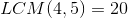.

The elements ofare precisely the mulitples of 20: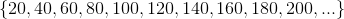All of the numbers end in 0 and have 2, 4, 6, 8,or 0 as their second-to-last digit.

Statement 1 does not, by itself, prove or disprove that, since there are numbers like 10 and 30 that do not fall in this set. But none of the elements of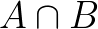have 5 as their second-to-last digit, so Statement 2 provesto be false.

### Example Question #7 : Sets

Two courses open to students in their senior year at Johnson High School are calculus and physics; students may take either or both. Of the 524 seniors enrolled at JHS, are more of them taking calculus or physics?

Statement 1: 139 students are taking neither course.

Statement 2: One-third of the students enrolled in calculus are also enrolled in physics.

BOTH statements TOGETHER are sufficient to answer the question, but NEITHER statement ALONE is sufficient to answer the question.

Statement 2 ALONE is sufficient to answer the question, but Statement 1 ALONE is NOT sufficient to answer the question.

BOTH statements TOGETHER are insufficient to answer the question.

EITHER statement ALONE is sufficient to answer the question.

Statement 1 ALONE is sufficient to answer the question, but Statement 2 ALONE is NOT sufficient to answer the question.

BOTH statements TOGETHER are insufficient to answer the question.

Explanation:

Suppose you know both statements. From the first statement, you can calculate that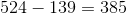seniors are taking calculus, physics, or both. However, as illustrated by these two examples, you cannot tell which course has more seniors enrolled.

Example 1: 50 seniors are enrolled in both courses.

Then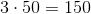seniors are enrolled in calculus;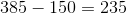seniors are enrolled in physics but not calculus;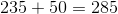seniors total are enrolled in physics. This means that seniors in physics outnumber seniors in calculus.

Example 2: 100 seniors are enrolled both courses.

Then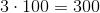seniors are enrolled in calculus;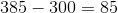seniors are enrolled in physics but not calculus;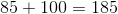seniors total are enrolled in physics. This means that seniors in calculus outnumber seniors in physics.

### Example Question #8 : Sets

Define sets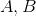as follows: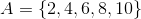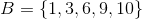What is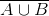?

Statement 1:Statement 2: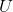comprises ten elements, all of which are positive integers.

Statement 2 ALONE is sufficient to answer the question, but Statement 1 ALONE is NOT sufficient to answer the question.

BOTH statements TOGETHER are sufficient to answer the question, but NEITHER statement ALONE is sufficient to answer the question.

Statement 1 ALONE is sufficient to answer the question, but Statement 2 ALONE is NOT sufficient to answer the question.

BOTH statements TOGETHER are insufficient to answer the question.

EITHER statement ALONE is sufficient to answer the question.

Statement 1 ALONE is sufficient to answer the question, but Statement 2 ALONE is NOT sufficient to answer the question.

Explanation: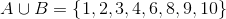is the complement of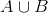- that is, the set of all elements in the universal setthat are not in. To findgiven, we need to know the elements in. Statement 1 gives us this information; Statement 2 does not.

### Example Question #9 : Sets

If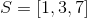, what is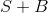?

(1)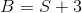(2)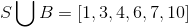EACH statement ALONE is sufficient

Statement (1) ALONE is sufficient, but statement (2) alone is not sufficient

BOTH statements TOGETHER are sufficient, but NEITHER statement ALONE is sufficient

Statement (2) ALONE is sufficient, but statement (1) alone is not sufficient

Statements (1) and (2) TOGETHER are not sufficient

Statement (1) ALONE is sufficient, but statement (2) alone is not sufficient

Explanation:

Statement (1) allows us to find B:

B = S + 3 = {4 , 6 , 10} Therefore:

S + B = {1+4 , 1+6 , 1+10 , 3+4 , 3+6 , 3+10 , 7+4 , 7+6 , 7+10}

S + B = {5 , 7 , 9 , 11 , 13 , 17}. SO statement (1) is sufficient to find S+B

Statement (2) does not give us enough information to find B. It could be any set between  {4 , 6 , 10} and {1 , 3 , 4 , 6 , 7 , 10} if it includes the same numbers as S. Therefore Statement 2 is not sufficient.

### Example Question #10 : Sets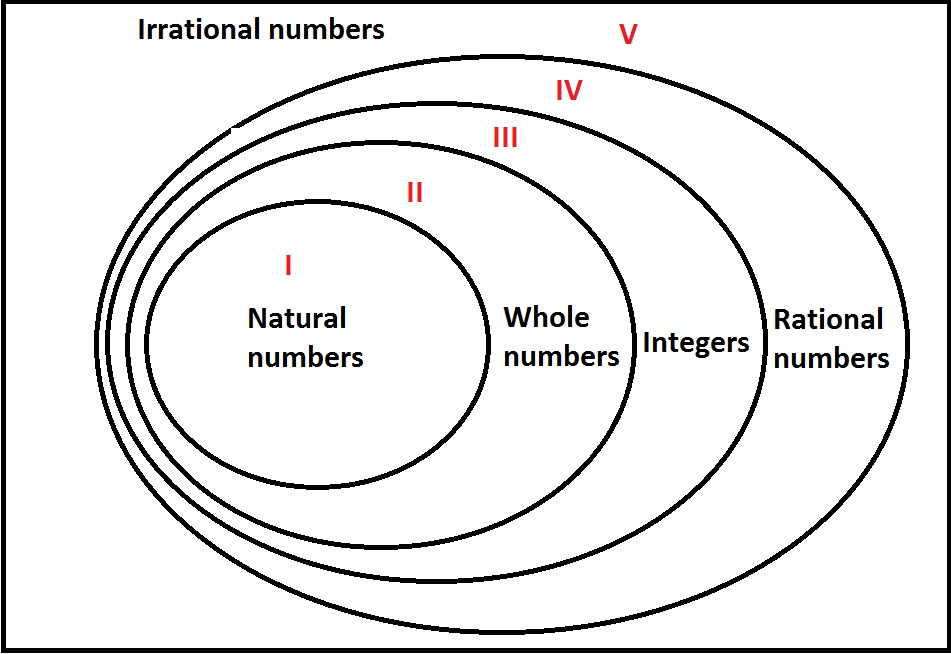Examine the above Venn diagram, which represents the sets of real numbers.

If real numberwere to be placed in its correct region in the diagram, which one would it be - I, II, III, IV, or V?

Statement 1:is negative.

Statement 2: If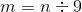, thenwould be placed in Region III.

EITHER statement ALONE is sufficient to answer the question.

Statement 1 ALONE is sufficient to answer the question, but Statement 2 ALONE is NOT sufficient to answer the question.

Statement 2 ALONE is sufficient to answer the question, but Statement 1 ALONE is NOT sufficient to answer the question.

BOTH statements TOGETHER are sufficient to answer the question, but NEITHER statement ALONE is sufficient to answer the question.

BOTH statements TOGETHER are insufficient to answer the question.

Statement 2 alone states thatis an integer that as not a whole number - that is,is a negative integer. Since, as a consequence,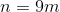, the product of a positive integer and a negative integer, is a negative integer, and it would be placed in Region III, which comprises exactly the negative integers.